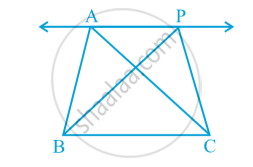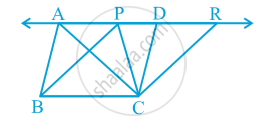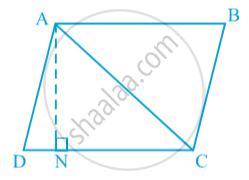# Corollary: Triangles on the same base and between the same parallels are equal in area.

#### notes

Two triangles ABC and PBC on the same base BC and between the same parallels BC and AP.  in following fig.Draw CD || BA and CR || BP such that D and R lie on line AP in following fig.From this, you obtain two parallelograms  PBCR and ABCD on the same base BC and between the same parallels BC and AR.
Therefore, ar (ABCD) = ar (PBCR)
Now ∆ ABC ≅ ∆ CDA and ∆ PBC ≅ ∆ CRP
So, ar (ABC) = 1/2 ar (ABCD) and ar (PBC) = 1/2 ar (PBCR)
Therefore, ar (ABC) = ar (PBC)

#### theorem

Theorem : Two triangles on the same base (or equal bases) and between the same parallels are equal in area.
Proof: Now, suppose ABCD is a parallelogram whose one of the diagonals is AC (see Fig.).Let AN ⊥ DC. Note that
So, ar (ADC) = ar (CBA)
Therefore, ar (ADC) = 1/2 ar (ABCD)
=1/2 (DC × AN)
So, area of ∆ ADC = 1/2 × base DC × corresponding altitude AN
In other words, area of a triangle is half the product of its base (or any side) and the corresponding altitude (or height).
The two triangles with same base (or equal bases) and equal areas will have equal corresponding altitudes.

If you would like to contribute notes or other learning material, please submit them using the button below.

### Shaalaa.com

Theorem : Two triangles on the same base (or equal bases) and between the same parallels are equal in area. [00:03:10]
S